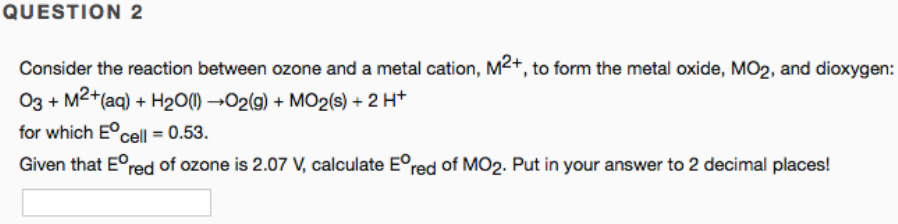# Consider the reaction between ozone and a metal cation, M^2+, to form the metal oxide, MO2, and dioxygen: O3 + M^2+(aq) + H2O(l) → O2(g) + MO2(s) + 2 H^+ for which E°cell = 0.53. Given that E°red of ozone is 2.07 V, calculate E°red of MO2. Put in your answer to 2 decimal places!